# Waveform Differentiation

A waveform is a function that repeats itself or cycles after a specified period of time. This time, denoted T, is called the period of the waveform.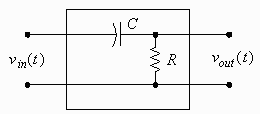The diagram to the right shows an electronic circuit that takes as input a voltage waveform and gives as output an approximation of the derivative of that waveform. The approximation can be made quite good by using small enough values of R and C or restricting ourselves to slowly varying input voltages.

To calculate the derivative of a waveform it is sufficient to calculate the derivative of just the first cycle. This is because the derivative of a waveform is another waveform. The derivative of all the other cycles will look the same as that of the first cycle. If f (t) is the formula for the first cycle then f ′ (t), its derivative, is the formula for the derivative of the first cycle. If required, the formula for the derivative of any other cycle can be found by simply applying a shift to the formula for the derivative of the first cycle.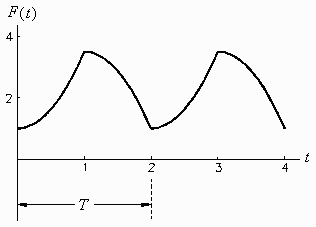## Problem

Calculate and sketch the derivative of the waveform shown to the right, whose period is 2 seconds and whose first cycle is defined as: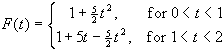## Solution

We begin by calculating the derivative, F′(t), of the above formula: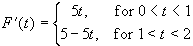This is the formula for the first cycle of the derivative. Any other cycle looks the same as the first one. A sketch of this derivative is shown below.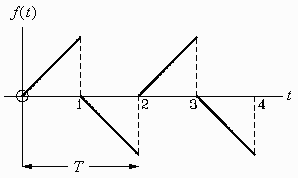# Maximum Power Transfer in a Circuit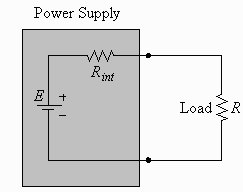## Problem

A power supply can be represented by an ideal voltage source of E volts in series with an internal resistance Rint. If a load is connected to the power supply, show that the maximum power that can be supplied to the load is achieved when the resistance R of the load is chosen to equal the internal resistance Rint of the power supply.

## Solution

We will express the power P dissipated in the resistor R as a function of R and then find where this function has its maximum. The power P dissipated in a resistor R is given by the formula P = I 2 R, where I is the current flowing through the resistor. Expressing the current I as a function of R (using Ohm’s law) gives: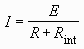Now we can express the power P as a function of R: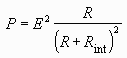Notice that this function is zero when R is zero, is positive for all positive values of R, and decreases like 1/R when R is very large. Clearly P must have a maximum. Now calculate the derivative dP/dR: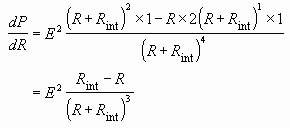This derivative is equal to zero when R = Rint. From the above discussion it is clear that the derivative is zero here because this is the value of the resistance R at which the power P is a maximum (as opposed to say a minimum).Written by Eric Hiob, September 27, 1997